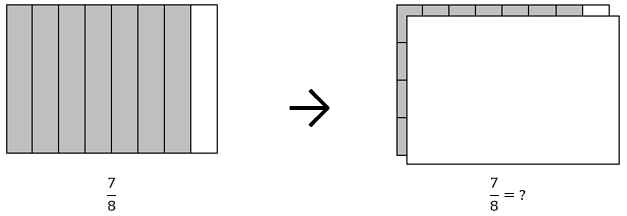# Fraction Equivalence and Ordering

## Objective

Recognize and generate equivalent fractions with smaller units using multiplication.

## Common Core Standards

### Core Standards

?

• 4.NF.A.1 — Explain why a fraction a/b is equivalent to a fraction (n × a)/(n × b) by using visual fraction models, with attention to how the number and size of the parts differ even though the two fractions themselves are the same size. Use this principle to recognize and generate equivalent fractions.

?

• 3.NF.A.3

• 4.OA.A.1

• 4.OA.A.2

## Criteria for Success

?

1. Develop a general method for finding equivalent fractions by multiplying both the numerator and the denominator by the same non-zero value.
2. Understand how the numbers and sizes of parts differ even though the two fractions are the same size, and connect this idea to the general method of using multiplication to find an equivalent fraction (MP.7).
3. Generate equivalent fractions with smaller units using the general method.
4. Determine whether two fractions are equivalent using multiplication, and support that work with a visual model (MP.3, MP.5).

## Tips for Teachers

?

As a supplement to the Problem Set, students can play a game to practice finding equivalent fractions with smaller units with increasing efficiency, such as “Fraction Scattergories”.

#### Fishtank Plus

• Problem Set
• Student Handout Editor
• Vocabulary Package

?

### Problem 1

Ms. Allen drew an area model to represent ${{7\over8}}$, as shown to the bottom left. She partitioned it to find an equivalent fraction, the covered model to the bottom right.What equivalent fraction did Ms. Allen come up with? How do you know?

#### References

John A. Van de Walle Teaching Student-Centered Mathematics: Developmentally Appropriate Instruction for Grades 3-5 (Volume II)Figure 12.19 (p. 225)

Van de Walle, John A. Teaching Student-Centered Mathematics: Developmentally Appropriate Instruction for Grades 3-5 (Volume II). Pearson, 2nd edition, 2013.

Modified by The Match Foundation, Inc.

### Problem 2

Find a fraction that is equivalent to each of the following:

a.   $\frac{1}{9}$

b.   ${{4\over7}}$

c.   ${{14\over11}}$

### Problem 3

Determine whether each of the following number sentences are true. Correct those that are false by changing either the numerator or denominator in the fraction on the right-hand side of the number sentence.

a.      ${{3\over4}={6\over12}}$

b.      ${{9\over5}={27\over15}}$

#### References

EngageNY Mathematics Grade 4 Mathematics > Module 5 > Topic B > Lesson 8Problem Set, Question #5

Grade 4 Mathematics > Module 5 > Topic B > Lesson 8 of the New York State Common Core Mathematics Curriculum from EngageNY and Great Minds. © 2015 Great Minds. Licensed by EngageNY of the New York State Education Department under the CC BY-NC-SA 3.0 US license. Accessed Dec. 2, 2016, 5:15 p.m..

Modified by The Match Foundation, Inc.

## Problem Set & Homework

#### Discussion of Problem Set

• Look at #2. How are the total number of fractional pieces and the total number of shaded fractional pieces related to the equation ${{2\times2}\over{3\times2}}$?
• Which number sentences were true in #6? How did you alter the false ones to make them true?
• What was Matilde’s error in #7?
• What were the missing values in #8?
• How did you solve #9? What did you need to remember about the angle measure of a complete circle?

?

### Problem 1

Find two fractions that are equivalent to ${{7\over12}}$ whose numerators are greater than $7$.

#### References

Achieve the Core Fraction Concepts Mini-AssessmentQuestion #6

Fraction Concepts Mini-Assessment by Student Achievement Partners is made available by Achieve the Core under the CC0 1.0 license. Accessed March 23, 2018, 9:16 a.m..

Modified by The Match Foundation, Inc.

### Problem 2

Determine if the following fractions are equivalent. Then explain how you know.

${{5\over3}={15\over9}}$

?